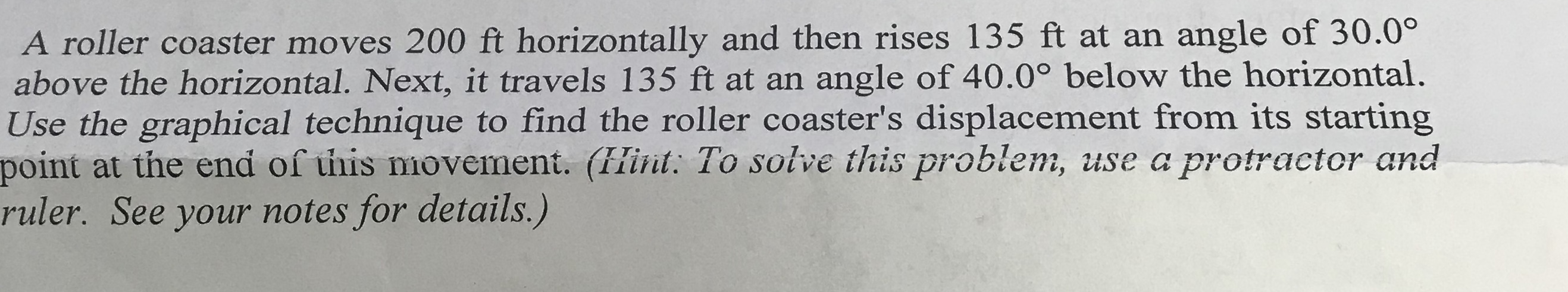# A roller coaster moves 200 ft horizontally and then rises 135 ft at an angle of 30.0°above the horizontal. Next, it travels 135 ft at an angle of 40.0° below the horizontal.Use the graphical technique to find the roller coaster's displacement from its startingpoint at the end of this movement. (fHint. To soive this problem, use a protractor andruler. See your notes for details.)

Question
230 views

I am not familiar with using protractors. So idk how to draw the diagram as well as what formulas to use. Thank you.help_outlineImage TranscriptioncloseA roller coaster moves 200 ft horizontally and then rises 135 ft at an angle of 30.0° above the horizontal. Next, it travels 135 ft at an angle of 40.0° below the horizontal. Use the graphical technique to find the roller coaster's displacement from its starting point at the end of this movement. (fHint. To soive this problem, use a protractor and ruler. See your notes for details.) fullscreen
check_circle

Step 1

The x component of the total displacement vector is,

Substituting the values, to find vector rx.

Step 2

The y component of the total displacement vector is,

Substituting the values, to find vector ry.

Step 3

The total displacement vector of the roller coaster is.

Sub...

### Want to see the full answer?

See Solution

#### Want to see this answer and more?

Solutions are written by subject experts who are available 24/7. Questions are typically answered within 1 hour.*

See Solution
*Response times may vary by subject and question.
Tagged in

### Vectors and Scalars